Do you like the article?
Share it with others -
post a link to it!
Use new possibilities of MetaTrader 5

# Graphical Interfaces V: The Vertical and Horizontal Scrollbar (Chapter 1)

16 May 2016, 15:46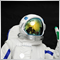1
8 695

### Introduction

In the previous articles of the series about graphical interfaces in the MetaTrader environment, we discussed the main parts of the library under development and also created several interface elements: the main menu, the context menu, the status bar, buttons, button groups and tooltips. The fifth part of the series will be dedicated the scrollbar and list view controls. In the first chapter, we will write classes for creating a vertical and horizontal scrollbar. In the second chapter, we will develop the list view — a compound interface element. It is compound because a scrollbar will be a part of it, so let us start with creating it.

### The Scrollbar Control

A scrollbar is used in different list views and tables when the data set does not fit a designated area. The main objects of the scrollbar are buttons for moving the data by one step and a slider for the quick movement of the data by dragging it with the left mouse button pressed down.

We will compose the scrollbar of five graphical objects.

1. Main background.
2. Background of the slider area.
3. Two buttons for moving data by one step.
4. Slider for the quick movement of the data.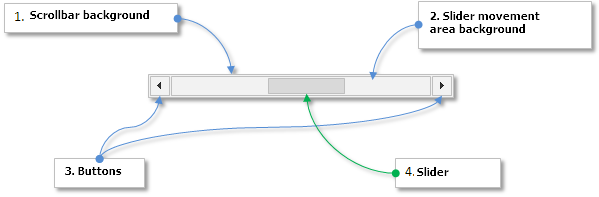Fig. 1. Compound parts of the scrollbar control.

As a scrollbar can be of two types - horizontal and vertical, it will be useful to make a separate class for each type. These will be derived classes for reflecting unique properties of each type of the scrollbar.

• CScrollV – derived class for the vertical scrollbar.
• CScrollH – derived class for the horizontal scrollbar.

The CScroll class will be the base class for them. It will contain fields and methods common for each type. All three classes will be placed in the Scrolls.mqh file. The schematic for the scrollbar control will look as shown below: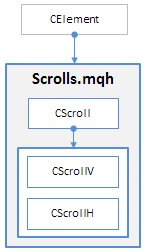Fig. 2. Schematic of the scrollbar control.

Now, we are going to consider the development of the base class for this control CScroll

### Base Class of the Control

The scrollbar control is not an independent element of graphical interface. It is an auxiliary element and it will be connected to other elements that require the scrolling of data in the working area. That means that the Scrolls.mqh file does not need to be directly included in the WndContainer.mqh file. The classes of the scrollbar will be available in the library through the inclusion in the files with classes of other elements.

Create the CScroll class in the Scrolls.mqh file with the standard methods for all interface elements:

```//+------------------------------------------------------------------+
//|                                                      Scrolls.mqh |
//|                        Copyright 2015, MetaQuotes Software Corp. |
//|                                              http://www.mql5.com |
//+------------------------------------------------------------------+
#include "Element.mqh"
#include "Window.mqh"
//+------------------------------------------------------------------+
//| Base class for creating the scrollbar                            |
//+------------------------------------------------------------------+
class CScroll : public CElement
{
protected:
//--- Pointer to the form to which the element is attached
CWindow          *m_wnd;
//---
public:
//--- Stores the form pointer
void              WindowPointer(CWindow &object)           { m_wnd=::GetPointer(object);       }
//---
public:
//--- Chart event handler
virtual void      OnEvent(const int id,const long &lparam,const double &dparam,const string &sparam);
//--- Timer
virtual void      OnEventTimer(void) {}
//--- Moving the element
virtual void      Moving(const int x,const int y);
//--- (1) Show, (2) hide, (3) reset, (4) delete
virtual void      Show(void);
virtual void      Hide(void);
virtual void      Reset(void);
virtual void      Delete(void);
//--- (1) Set, (2) reset priorities of the left mouse button click
virtual void      SetZorders(void);
virtual void      ResetZorders(void);
//--- Reset color
virtual void      ResetColors(void) {}
};
//+------------------------------------------------------------------+
//| Constructor                                                      |
//+------------------------------------------------------------------+
CScroll::CScroll(void)
{
}
//+------------------------------------------------------------------+
//| Destructor                                                       |
//+------------------------------------------------------------------+
CScroll::~CScroll(void)
{
}
```

Skipping ahead, it should be mentioned that derived classes must be created in the same file as shown in the code below. Depending on which class is going to be used, the element gets a corresponding name in the class constructor.

```//+------------------------------------------------------------------+
//| Class for managing the vertical scrollbar                        |
//+------------------------------------------------------------------+
class CScrollV : public CScroll
{
public:
CScrollV(void);
~CScrollV(void);
};
//+------------------------------------------------------------------+
//| Constructor                                                      |
//+------------------------------------------------------------------+
CScrollV::CScrollV(void)
{
//--- Store the name of the element class in the base class
CElement::ClassName(CLASS_NAME);
}
//+------------------------------------------------------------------+
//| Destructor                                                       |
//+------------------------------------------------------------------+
CScrollV::~CScrollV(void)
{
}
//+------------------------------------------------------------------+
//| Class for managing the horizontal scrollbar                      |
//+------------------------------------------------------------------+
class CScrollH : public CScroll
{
public:
CScrollH(void);
~CScrollH(void);
};
//+------------------------------------------------------------------+
//| Constructor                                                      |
//+------------------------------------------------------------------+
CScrollH::CScrollH(void)
{
//--- Store the name of the element class in the base class
CElement::ClassName(CLASS_NAME);
}
//+------------------------------------------------------------------+
//| Destructor                                                       |
//+------------------------------------------------------------------+
CScrollH::~CScrollH(void)
{
}
```

As mentioned earlier, let us enable the customization of the scrollbar appearance. For that, in the base class CScroll create fields and methods for setting the following parameters of the element objects:

• Width of the scrollbar
• Color of the general background frame
• Color of the background and the frame of the area where the slider of the scrollbar is located
• Color of the background and the frame of the slider in different states
• Icons for different states of the buttons, with which data will be scrolled

It must be noted that the width of the vertical scrollbar and its objects will be the size along the X axis and the width of horizontal scrollbar will be the size along the Y axis. The default width will be 15 pixels. The default icons for the buttons are selected to match this size. They are located inside the general background with the margin of 1 pixel so that they do not cover the background frame. Their size is 13x13 pixels. Therefore, if you decide to change the width of the scrollbar, you will need to change the button icons. Let us create relevant methods.

The width of other objects of the scrollbar (internal area and the slider) will be calculated automatically in relation to the width of the general background. The length will also be calculated automatically as this parameter depends on the number of items in the list and their size along the Y axis. The implementation will be described further in the article.

```class CScroll : public CElement
{
protected:
//--- Properties of the general area of the scrollbar
int               m_area_width;
int               m_area_length;
color             m_area_color;
color             m_area_border_color;
//--- Properties of the slider background
int               m_bg_length;
color             m_bg_border_color;
//--- Button icons
string            m_inc_file_on;
string            m_inc_file_off;
string            m_dec_file_on;
string            m_dec_file_off;
//--- Colors of the slider in different states
color             m_thumb_color;
color             m_thumb_color_hover;
color             m_thumb_color_pressed;
color             m_thumb_border_color;
color             m_thumb_border_color_hover;
color             m_thumb_border_color_pressed;
//--- (1) Width of the slider, (2) length of the slider (3) and its minimal length
int               m_thumb_width;
int               m_thumb_length;
int               m_thumb_min_length;
//--- (1) Step of the slider and (2) the number of steps
double            m_thumb_step_size;
double            m_thumb_steps_total;
//--- Priorities of the left mouse button click
int               m_area_zorder;
int               m_bg_zorder;
int               m_arrow_zorder;
int               m_thumb_zorder;
//---
public:
//--- Width of the slider
void              ScrollWidth(const int width)             { m_area_width=width;               }
int               ScrollWidth(void)                  const { return(m_area_width);             }
//--- (1) Color of the background, (2) of the background frame and (3) the internal frame of the background
void              AreaColor(const color clr)               { m_area_color=clr;                 }
void              AreaBorderColor(const color clr)         { m_area_border_color=clr;          }
void              BgBorderColor(const color clr)           { m_bg_border_color=clr;            }
//--- Setting icons for buttons
void              IncFileOn(const string file_path)        { m_inc_file_on=file_path;          }
void              IncFileOff(const string file_path)       { m_inc_file_off=file_path;         }
void              DecFileOn(const string file_path)        { m_dec_file_on=file_path;          }
void              DecFileOff(const string file_path)       { m_dec_file_off=file_path;         }
//--- (1) Color of the slider background and (2) the frame of the slider background
void              ThumbColor(const color clr)              { m_thumb_border_color=clr;         }
void              ThumbColorHover(const color clr)         { m_thumb_border_color_hover=clr;   }
void              ThumbColorPressed(const color clr)       { m_thumb_border_color_pressed=clr; }
void              ThumbBorderColor(const color clr)        { m_thumb_border_color=clr;         }
void              ThumbBorderColorHover(const color clr)   { m_thumb_border_color_hover=clr;   }
void              ThumbBorderColorPressed(const color clr) { m_thumb_border_color_pressed=clr; }
};
//+------------------------------------------------------------------+
//| Constructor                                                      |
//+------------------------------------------------------------------+
CScroll::CScroll(void) : m_area_width(15),
m_area_length(0),
m_inc_file_on(""),
m_inc_file_off(""),
m_dec_file_on(""),
m_dec_file_off(""),
m_thumb_width(0),
m_thumb_length(0),
m_thumb_min_length(15),
m_area_color(C'210,210,210'),
m_area_border_color(C'240,240,240'),
m_bg_border_color(C'210,210,210'),
m_thumb_color(C'190,190,190'),
m_thumb_color_hover(C'180,180,180'),
m_thumb_color_pressed(C'160,160,160'),
m_thumb_border_color(C'170,170,170'),
m_thumb_border_color_hover(C'160,160,160'),
m_thumb_border_color_pressed(C'140,140,140')
{
//--- Set priorities of the left mouse button click
m_area_zorder  =8;
m_bg_zorder    =9;
m_arrow_zorder =10;
m_thumb_zorder =11;
}
```

Let us consider methods for creating the scrollbar element. Each part of it is created by a separate private method. The size of the list view and the size of the visible part of the list view must be passed as the last two parameters in the main method. These parameters will then be used in the calculation of the number of steps for the scrollbar slider.

```class CScroll : public CElement
{
protected:
//--- Objects for creating the scrollbar
CRectLabel        m_area;
CRectLabel        m_bg;
CBmpLabel         m_inc;
CBmpLabel         m_dec;
CRectLabel        m_thumb;
//---
public:
//--- Methods for creating the scrollbar
bool              CreateScroll(const long chart_id,const int subwin,const int x,const int y,const int items_total,const int visible_items_total);
//---
private:
bool              CreateArea(void);
bool              CreateBg(void);
bool              CreateInc(void);
bool              CreateDec(void);
bool              CreateThumb(void);
};
```

We are going to show the code of only some of these methods as an example because similar principles are used in the rest of the methods, which should be easy to follow. Take a look at the code of the CScroll::CreateBg() method below. Please note, that the formation of the object name depends on the scrollbar type and also on whether the index of this element is specified. Specifying the index may be required in the development of compound controls where several scrollbars of one type may be used. Such examples will be illustrated in one of the following articles.

The scrollbar type also defines the calculations of such parameters as (1) coordinates, (2) length of the slider area and (3) its size. This part is highlighted in blue in the code below.

```//+------------------------------------------------------------------+
//| Creates the scrollbar background                                 |
//+------------------------------------------------------------------+
bool CScroll::CreateBg(void)
{
//--- Forming the object name
string name      ="";
string name_part =(CElement::ClassName()=="CScrollV")? "_scrollv_bg_" : "_scrollh_bg_";
//--- If the index has not been specified
if(CElement::Index()==WRONG_VALUE)
name=CElement::ProgramName()+name_part+(string)CElement::Id();
//--- If the index has been specified
else
name=CElement::ProgramName()+name_part+(string)CElement::Index()+"__"+(string)CElement::Id();
//--- Coordinates
int x=0;
int y=0;
//--- Size
int x_size=0;
int y_size=0;
//--- Setting properties considering the scrollbar type
if(CElement::ClassName()=="CScrollV")
{
m_bg_length =CElement::YSize()-(m_thumb_width*2)-2;
x           =CElement::X()+1;
y           =CElement::Y()+m_thumb_width+1;
x_size      =m_thumb_width;
y_size      =m_bg_length;
}
else
{
m_bg_length =CElement::XSize()-(m_thumb_width*2)-2;
x           =CElement::X()+m_thumb_width+1;
y           =CElement::Y()+1;
x_size      =m_bg_length;
y_size      =m_thumb_width;
}
//--- Creating an object
if(!m_bg.Create(m_chart_id,name,m_subwin,x,y,x_size,y_size))
return(false);
//--- Setting properties
m_bg.BackColor(m_area_color);
m_bg.Color(m_bg_border_color);
m_bg.BorderType(BORDER_FLAT);
m_bg.Corner(m_corner);
m_bg.Selectable(false);
m_bg.Z_Order(m_bg_zorder);
m_bg.Tooltip("\n");
//--- Store coordinates
m_bg.X(x);
m_bg.Y(y);
//--- Store margins
m_bg.XGap(x-m_wnd.X());
m_bg.YGap(y-m_wnd.Y());
//--- Store the size
m_bg.XSize(x_size);
m_bg.YSize(y_size);
//--- Store the object pointer
CElement::AddToArray(m_bg);
return(true);
}
```

When creating the graphical object that will act as the slider of the scrollbar, we will need a method for calculating its length. The length depends on the number of items in the list view and on the number of items in its visible part. Let us create such a method and call it CScroll::CalculateThumbSize().

At the beginning of this method there is a check for the size of the slider area. If the length of this area is less than a minimal length of the slider, then the calculation is not needed. The method will return false and a slider will not be created as it is not needed in such case. If the check is passed, then the calculation will have several stages:

• Calculation of the slider step.
• If the obtained value is less than 1, then make it equal to 1.
• Calculation of the working area for moving the slider.
• If the size of the working area is less than the size of the whole area of moving the slider, then we calculate the length of the slider. Otherwise, set a minimal length by default equal to 15 pixels.
• The check of the calculated length of the slider, which involves type casting to the integer type (int) takes place at the end of the method as well as the adjustment in case the calculated length is less than the minimal one.

Detailed code of the CScroll::CalculateThumbSize() method is shown below.

```class CScroll : public CElement
{
protected:

public:
//--- Calculation of the length of the scrollbar slider
bool              CalculateThumbSize(void);
};
//+------------------------------------------------------------------+
//| Calculation of the length of the scrollbar slider                |
//+------------------------------------------------------------------+
bool CScroll::CalculateThumbSize(void)
{
//--- Calculation is not required if the length of the area for moving the slider is less than the minimal length of the slider
if(m_bg_length<m_thumb_min_length)
return(false);
//--- Calculate the size of the slider step
m_thumb_step_size=(double)(m_bg_length-m_thumb_min_length)/m_thumb_steps_total;
//--- The step size cannot be less than 1
m_thumb_step_size=(m_thumb_step_size<1)? 1 : m_thumb_step_size;
//--- Calculate the size of the working area for moving the slider
double work_area=m_thumb_step_size*m_thumb_steps_total;
//--- If the size of the working area is less than the size of the whole area, get the size of the slider otherwise set the minimal size
double thumb_size=(work_area<m_bg_length)? m_bg_length-work_area+m_thumb_step_size : m_thumb_min_length;
//--- Check of the slider size using the type casting
m_thumb_length=((int)thumb_size<m_thumb_min_length)? m_thumb_min_length :(int)thumb_size;
return(true);
}
```

The call of the CScroll::CalculateThumbSize() method must be carried out in the CScroll::CreateThumb() method before creating the object. Later, we will illustrate another case when the length of the scrollbar must be calculated in the process of using the control. We will consider it when we are developing the control.

```//+------------------------------------------------------------------+
//| Creates the slider of the scrollbar                              |
//+------------------------------------------------------------------+
bool CScroll::CreateThumb(void)
{
//--- Forming the object name
string name      ="";
string name_part =(CElement::ClassName()=="CScrollV")? "_scrollv_thumb_" : "_scrollh_thumb_";
//--- If the index has not been specified
if(CElement::Index()==WRONG_VALUE)
name=CElement::ProgramName()+name_part+(string)CElement::Id();
//--- If the index has been specified
else
name=CElement::ProgramName()+name_part+(string)CElement::Index()+"__"+(string)CElement::Id();
//--- Coordinates
int x=0;
int y=0;
//--- Size
int x_size=0;
int y_size=0;
//--- Calculate the size of the scrollbar
if(!CalculateThumbSize())
return(true);
//--- Setting the property considering the scrollbar type
if(CElement::ClassName()=="CScrollV")
{
x      =(m_thumb.X()>0) ? m_thumb.X() : m_x+1;
y      =(m_thumb.Y()>0) ? m_thumb.Y() : m_y+m_thumb_width+1;
x_size =m_thumb_width;
y_size =m_thumb_length;
}
else
{
x      =(m_thumb.X()>0) ? m_thumb.X() : m_x+m_thumb_width+1;
y      =(m_thumb.Y()>0) ? m_thumb.Y() : m_y+1;
x_size =m_thumb_length;
y_size =m_thumb_width;
}
//--- Creating an object
if(!m_thumb.Create(m_chart_id,name,m_subwin,x,y,x_size,y_size))
return(false);
//--- Setting properties
m_thumb.BackColor(m_thumb_color);
m_thumb.Color(m_thumb_border_color);
m_thumb.BorderType(BORDER_FLAT);
m_thumb.Corner(m_corner);
m_thumb.Selectable(false);
m_thumb.Z_Order(m_thumb_zorder);
m_thumb.Tooltip("\n");
//--- Store coordinates
m_thumb.X(x);
m_thumb.Y(y);
//--- Store margins
m_thumb.XGap(x-m_wnd.X());
m_thumb.YGap(y-m_wnd.Y());
//--- Store the size
m_thumb.XSize(x_size);
m_thumb.YSize(y_size);
//--- Store the object pointer
CElement::AddToArray(m_thumb);
return(true);
}
```

Other methods for creating the scrollbar objects are meant for individual study. They can be found in the file attached to this article.

Add the check for the class type in the main (public) method for creating the scrollbar. The name of the class will not be stored in the base class of the element CScroll. That is why, when attempting to create a scrollbar using the base class, the creation of the graphical interface will be terminated. The journal will receive a message that the derived classes of the CScrollV or CScrollH type must be used. Please note how some parameters of the element are initialized. Such approach allows to make all the calculations automated to a great extent sparing library users a routine and providing the possibility to specify a minimal number of properties when creating controls with a scrollbar.

```//+------------------------------------------------------------------+
//| Creates the scrollbar                                            |
//+------------------------------------------------------------------+
bool CScroll::CreateScroll(const long chart_id,const int subwin,const int x,const int y,const int items_total,const int visible_items_total)
{
//--- Leave, if there is no form pointer
if(::CheckPointer(m_wnd)==POINTER_INVALID)
{
::Print(__FUNCTION__," > Before creating the scrollbar, the class must be passed "
"the form pointer: CScroll::WindowPointer(CWindow &object)");
return(false);
}
//--- Leave, if there is an attempt to use the base class of the scrollbar
if(CElement::ClassName()=="")
{
::Print(__FUNCTION__," > Use derived classes of the scrollbar (CScrollV or CScrollH).");
return(false);
}
//--- Initializing variables
m_chart_id          =chart_id;
m_subwin            =subwin;
m_x                 =x;
m_y                 =y;
m_area_width        =(CElement::ClassName()=="CScrollV")? CElement::XSize() : CElement::YSize();
m_area_length       =(CElement::ClassName()=="CScrollV")? CElement::YSize() : CElement::XSize();
m_thumb_width       =m_area_width-2;
m_thumb_steps_total =items_total-visible_items_total+1;
//--- Margins from the edge
CElement::XGap(m_x-m_wnd.X());
CElement::YGap(m_y-m_wnd.Y());
//--- Creating the button
if(!CreateArea())
return(false);
if(!CreateBg())
return(false);
if(!CreateInc())
return(false);
if(!CreateDec())
return(false);
if(!CreateThumb())
return(false);
//--- Hide the element if this is a dialog window or it is minimized
if(m_wnd.WindowType()==W_DIALOG || m_wnd.IsMinimized())
Hide();
//---
return(true);
}
```

To identify the state of the left mouse button in relation to the slider of the scrollbar, create the ENUM_THUMB_MOUSE_STATE enumeration in the Enums.mqh file.

```//+------------------------------------------------------------------+
//| Enumeration of states of the left mouse button for the scrollbar |
//+------------------------------------------------------------------+
enum ENUM_THUMB_MOUSE_STATE
{
THUMB_NOT_PRESSED     =0,
THUMB_PRESSED_OUTSIDE =1,
THUMB_PRESSED_INSIDE  =2
};
```

For that, we need to create appropriate fields and methods. The CScroll::CheckMouseButtonState() method is required for identifying over what area the left mouse button was pressed. The CScroll::ZeroThumbVariables() method is used for zeroing variables connected with moving the slider and is called in the CScroll::CheckMouseButtonState() method by the condition when the left mouse button is released.

Bear in mind, that when the scrollbar is in the mode of moving the slider, the form becomes blocked only if the element is not a drop-down. This is required to exclude highlighting other elements when the cursor is hovering over them, the slider of the scrollbar is in the movement mode and the cursor out of its area. When the movement mode of the slider is disabled, the form must be unblocked in the CScroll::ZeroThumbVariables() method

```class CScroll : public CElement
{
protected:
//--- Variables connected with the slider movement
bool              m_scroll_state;
int               m_thumb_size_fixing;
int               m_thumb_point_fixing;
//--- To identify the area of pressing down the left mouse button
ENUM_THUMB_MOUSE_STATE m_clamping_area_mouse;
//---
public:
//--- Identifies the area of pressing down the left mouse button
void              CheckMouseButtonState(const bool mouse_state);
//--- Zeroing variables
void              ZeroThumbVariables(void);
};
//+------------------------------------------------------------------+
//| Identifies the area of pressing the left mouse button            |
//+------------------------------------------------------------------+
void CScroll::CheckMouseButtonState(const bool mouse_state)
{
//--- Identify the state of the mouse button:
//    If released
if(!mouse_state)
{
//--- Zero variables
ZeroThumbVariables();
return;
}
//--- If pressed down
if(mouse_state)
{
//--- Leave, if the button is pressed down in another area
if(m_clamping_area_mouse!=THUMB_NOT_PRESSED)
return;
//--- Outside of the slider area
if(!m_thumb.MouseFocus())
m_clamping_area_mouse=THUMB_PRESSED_OUTSIDE;
//--- Inside the slider area
else
{
m_clamping_area_mouse=THUMB_PRESSED_INSIDE;
//--- If this is not a drop-down element
if(!CElement::IsDropdown())
{
//--- Block the form and store the active element identifier
m_wnd.IsLocked(true);
m_wnd.IdActivatedElement(CElement::Id());
}
}
}
}
//+------------------------------------------------------------------+
//| Zeroing variables connected with the slider movement             |
//+------------------------------------------------------------------+
void CScroll::ZeroThumbVariables(void)
{
//--- If this is not a drop-down element
if(!CElement::IsDropdown())
{
//--- Unblock the form and reset the active element identifier
m_wnd.IsLocked(false);
m_wnd.IdActivatedElement(WRONG_VALUE);
}
m_thumb_size_fixing   =0;
m_clamping_area_mouse =THUMB_NOT_PRESSED;
}
```

To change the color of the scrollbar objects depending on the mouse cursor location and the state of its left button, create the CScroll::ChangeObjectsColor() method. At the beginning of this method, there is a check if the form is blocked and if the scrollbar identifier differs from the identifier stored in the form memory. If both of these conditions are met, then depending on the current mode of the scrollbar and the focus over its buttons, they get a corresponding state. A scrollbar can have two modes: (1) free and (2) in the process of moving the slider. After that, depending on the cursor location and in what area the left mouse button was pressed down, the slider gets a relevant color. The mode of the scrollbar is defined here as well.

```class CScroll : public CElement
{
public:
//--- Changing the color of the scrollbar objects
void              ChangeObjectsColor(void);
};
//+------------------------------------------------------------------+
//| Changes the color of objects of the list view scrollbar          |
//+------------------------------------------------------------------+
void CScroll::ChangeObjectsColor(void)
{
//--- Leave, if the form is blocked and the identifier of the currently active element differs
if(m_wnd.IsLocked() && m_wnd.IdActivatedElement()!=CElement::Id())
return;
//--- Color of the buttons of the list view scrollbar
if(!m_scroll_state)
{
m_inc.State(m_inc.MouseFocus());
m_dec.State(m_dec.MouseFocus());
}
//--- If the cursor is in the scrollbar area
if(m_thumb.MouseFocus())
{
//--- If the left mouse button is released
if(m_clamping_area_mouse==THUMB_NOT_PRESSED)
{
m_scroll_state=false;
m_thumb.BackColor(m_thumb_color_hover);
m_thumb.Color(m_thumb_border_color_hover);
}
//--- The left mouse button is pressed down on the slider
else if(m_clamping_area_mouse==THUMB_PRESSED_INSIDE)
{
m_scroll_state=true;
m_thumb.BackColor(m_thumb_color_pressed);
m_thumb.Color(m_thumb_border_color_pressed);
}
}
//--- If the cursor is outside of the scrollbar area
else
{
//--- Left mouse button is released
if(m_clamping_area_mouse==THUMB_NOT_PRESSED)
{
m_scroll_state=false;
m_thumb.BackColor(m_thumb_color);
m_thumb.Color(m_thumb_border_color);
}
}
}
```

Methods for obtaining names and states of the scrollbar buttons will be required for the development of the interface elements with a scrollbar. In addition to that, we will need methods for setting and identifying the state of moving the slider of the scrollbar and its current location in relation to the list view to which it is attached.

```class CScroll : public CElement
{
protected:
//--- Defining the state of the scrollbar
bool              m_scroll_state;
//--- Current location of the slider
int               m_current_pos;
//---
public:
//--- Names of button objects
string            ScrollIncName(void)                const { return(m_inc.Name());             }
string            ScrollDecName(void)                const { return(m_dec.Name());             }
//--- State of buttons
bool              ScrollIncState(void)               const { return(m_inc.State());            }
bool              ScrollDecState(void)               const { return(m_dec.State());            }
//--- State of the scrollbar
void              ScrollState(const bool scroll_state)     { m_scroll_state=scroll_state;      }
bool              ScrollState(void)                  const { return(m_scroll_state);           }
//--- Current location of the slider
void              CurrentPos(const int pos)                { m_current_pos=pos;                }
int               CurrentPos(void)                   const { return(m_current_pos);            }
};
```

We have completed the development of the base class of the scrollbar. Now, we are going to fill derived classes of this control.

### Derived Classes of the Control

Whereas base class of the scrollbar is filled with general methods for creating objects, setting parameters and getting their values, derived classes are intended for managing this control. We have previously created derived classes CScrollV and CScrollH in the Scrolls.mqh file. We are going to discuss methods of only one of them - class of the vertical scrollbar (CScrollV). In the second class (CScrollH) everything is going to be the same with only one adjustment for scrollbar type. In the class of horizontal scrollbar we will be working with the X coordinate unlike the class of the vertical scrollbar where we work with the Y coordinate.

At first, we are going to consider the code of the CScrollV::OnDragThumb() and CScrollV::UpdateThumb() methods designed for moving the slider of the scrollbar. They have to be declared in the private section of the class as they will be used only inside the CScrollV class.

```//+------------------------------------------------------------------+
//| Class for managing the vertical scrollbar                        |
//+------------------------------------------------------------------+
class CScrollV : public CScroll
{
private:
//--- Moving the slider
void              OnDragThumb(const int y);
//--- Updating the location of the slider
void              UpdateThumb(const int new_y_point);
};
```

The CScrollV::OnDragThumb() method is required for identifying the user's attempt to move the slider. If the left mouse button was pressed down over the slider of the scrollbar and the cursor was moved in that state along the Y axis, it means that the process of movement has started and the object has to be moved. Updating of the slider coordinates is carried out with the CScrollV::UpdateThumb().

```//+------------------------------------------------------------------+
//| Moving the slider                                                |
//+------------------------------------------------------------------+
void CScrollV::OnDragThumb(const int y)
{
//--- To identify the new Y coordinate
int new_y_point=0;
//--- If the scrollbar is inactive, ...
if(!CScroll::ScrollState())
{
//--- ...zero auxiliary variables for moving the slider
CScroll::m_thumb_size_fixing  =0;
CScroll::m_thumb_point_fixing =0;
return;
}
//--- If the fixation point is zero, store current coordinates of the cursor
if(CScroll::m_thumb_point_fixing==0)
CScroll::m_thumb_point_fixing=y;
//--- If the distance from the edge of the slider to the current coordinate of the cursor is zero, calculate it
if(CScroll::m_thumb_size_fixing==0)
CScroll::m_thumb_size_fixing=m_thumb.Y()-y;
//--- If the threshold is passed downwards in the pressed down state
if(y-CScroll::m_thumb_point_fixing>0)
{
//--- Calculate the Y coordinate
new_y_point=y+CScroll::m_thumb_size_fixing;
//--- Update location of the slider
UpdateThumb(new_y_point);
return;
}
//--- If the threshold is passed upwards in the pressed down state
if(y-CScroll::m_thumb_point_fixing<0)
{
//--- Calculate the Y coordinate
new_y_point=y-::fabs(CScroll::m_thumb_size_fixing);
//--- Update location of the slider
UpdateThumb(new_y_point);
return;
}
}
```

Below is the detailed code of the CScrollV::UpdateThumb() method. The Y coordinate calculated in the CScrollV::OnDragThumb() method is passed to it. There it is checked for correctness. If it turns out that we exceed the limits of the working area designated for moving the slider, the values are adjusted. Only after that, coordinates are updated and stored in the fields of base classes.

```//+------------------------------------------------------------------+
//| Updating of the location of the slider                           |
//+------------------------------------------------------------------+
void CScrollV::UpdateThumb(const int new_y_point)
{
int y=new_y_point;
//--- Zeroing the fixation point
CScroll::m_thumb_point_fixing=0;
//--- Checking for exceeding the working area downwards and adjusting values
if(new_y_point>m_bg.Y2()-CScroll::m_thumb_length)
{
y=m_bg.Y2()-CScroll::m_thumb_length;
CScroll::CurrentPos(int(CScroll::m_thumb_steps_total));
}
//--- Checking for exceeding the working area upwards and adjusting values
if(new_y_point<=m_bg.Y())
{
y=m_bg.Y();
CScroll::CurrentPos(0);
}
//--- Update coordinates and margins
m_thumb.Y(y);
m_thumb.Y_Distance(y);
m_thumb.YGap(m_thumb.Y()-(CElement::Y()-CElement::YGap()));
}
```

We will need one more private method for correcting current position of the slider in relation to its Y coordinate. Let us name it CScrollV::CalculateThumbPos(). Its code is presented below.

```class CScrollV : public CScroll
{
private:
//--- Corrects the value of the slider position
void              CalculateThumbPos(void);
};
//+------------------------------------------------------------------+
//| Corrects the value of the slider position                        |
//+------------------------------------------------------------------+
void CScrollV::CalculateThumbPos(void)
{
//--- Leave, if the step is zero
if(CScroll::m_thumb_step_size==0)
return;
//--- Corrects the value of the position of the scrollbar
CScroll::CurrentPos(int((m_thumb.Y()-m_bg.Y())/CScroll::m_thumb_step_size));
//--- Check for exceeding the working area downwards/upwards
if(m_thumb.Y2()>=m_bg.Y2()-1)
CScroll::CurrentPos(int(CScroll::m_thumb_steps_total-1));
if(m_thumb.Y()<m_bg.Y())
CScroll::CurrentPos(0);
}
```

Now, let us create the CScrollV::ScrollBarControl() public method for managing the slider. All private methods considered above will be called in this method. It will have to be called in the OnEvent() event handlers of those elements containing a scrollbar. We will bring a detailed example in the second chapter where we will develop a class for creating the list view control.

The code of the CScrollV::ScrollBarControl() method is presented below. Cursor coordinates and the state of the left mouse button are passed to it as arguments. At the beginning, we check the focus over the scrollbar. Then, we check over what area the left mouse button was pressed down. Depending on the cursor location and the state of the left mouse button, the color of the scrollbar is changed. After that, if the management is passed to the scrollbar, the scrollbar is moved according to the new calculated Y coordinate. The number of the list view item is calculated in relation to it.

```class CScrollV : public CScroll
{
public:
//--- Managing the scrollbar
bool              ScrollBarControl(const int x,const int y,const bool mouse_state);
};
//+------------------------------------------------------------------+
//| Managing the scrollbar                                           |
//+------------------------------------------------------------------+
bool CScrollV::ScrollBarControl(const int x,const int y,const bool mouse_state)
{
//--- Checking the focus over the scrollbar
m_thumb.MouseFocus(x>m_thumb.X() && x<m_thumb.X2() &&
y>m_thumb.Y() && y<m_thumb.Y2());
//--- Check and store the state of the mouse button
CScroll::CheckMouseButtonState(mouse_state);
//--- Change the color of the scrollbar
CScroll::ChangeObjectsColor();
//--- If the management is passed to the scrollbar, identify the location of the scrollbar
if(CScroll::ScrollState())
{
//--- Moving the slider
OnDragThumb(y);
//--- Changes the value of the scrollbar location
CalculateThumbPos();
return(true);
}
//---
return(false);
}
```

The CScrollV::CalculateThumbPos() method converts the Y coordinate into the number of the list view item. We also need a method for making a reverse conversion, that is of calculating the Y coordinate in relation to the current position of the scrollbar. Here, there are also checks for exceeding the working area and adjustment in case of exceeding. At the end of the method, coordinates and margins of the object are updated.

```class CScrollV : public CScroll
{
public:
//--- Calculation of the Y coordinate of the scrollbar
void              CalculateThumbY(void);
};
//+------------------------------------------------------------------+
//| Calculation of the Y coordinate of the scrollbar                 |
//+------------------------------------------------------------------+
void CScrollV::CalculateThumbY(void)
{
//--- Identify current Y coordinate of the scrollbar
int scroll_thumb_y=int(m_bg.Y()+(CScroll::CurrentPos()*CScroll::m_thumb_step_size));
//--- If the working area is exceeded upwards
if(scroll_thumb_y<=m_bg.Y())
scroll_thumb_y=m_bg.Y();
//--- If the working area is exceeded downwards
if(scroll_thumb_y+CScroll::m_thumb_length>=m_bg.Y2() ||
CScroll::CurrentPos()>=CScroll::m_thumb_steps_total-1)
{
scroll_thumb_y=int(m_bg.Y2()-CScroll::m_thumb_length);
}
//--- Update the coordinate and margin along the Y axis
m_thumb.Y(scroll_thumb_y);
m_thumb.Y_Distance(scroll_thumb_y);
m_thumb.YGap(m_thumb.Y()-m_wnd.Y());
}
```

The CScrollV::CalculateThumbY() method will be called in the methods for handling of pressing on the scrollbar buttons as shown in the code below. The name of the object is the only argument that is passed to the CScrollV::OnClickScrollInc() and CScrollV::OnClickScrollDec() methods. The following parameters are checked at the very beginning of the method.

• If this button was pressed. Check by the object name.
• State of the scrollbar. For the check to be passed, the mode must be free.
• The number of steps must be defined and this value cannot be less than one.

If all the checks are passed, then the position is changed by one step considering that the working range cannot be exceeded. After that, in the CScrollV::CalculateThumbY() method the Y coordinate is calculated and updated as well as margins from the edge of the form. The button must stay in the on state after it was pressed down.

```class CScrollV : public CScroll
{
public:
//--- Handling the pressing on the scrollbar buttons
bool              OnClickScrollInc(const string clicked_object);
bool              OnClickScrollDec(const string clicked_object);
};
//+------------------------------------------------------------------+
//| Handling the pressing on the upwards/to the left button          |
//+------------------------------------------------------------------+
bool CScrollV::OnClickScrollInc(const string clicked_object)
{
//--- Leave, if the pressing was not on this object or the scrollbar is inactive or the number of steps is not identified
if(m_inc.Name()!=clicked_object || CScroll::ScrollState() || CScroll::m_thumb_steps_total<1)
return(false);
//--- Decrease the value of the scrollbar position
if(CScroll::CurrentPos()>0)
CScroll::m_current_pos--;
//--- Calculation of the Y coordinate of the scrollbar
CalculateThumbY();
//--- Set the On state
m_inc.State(true);
return(true);
}
//+------------------------------------------------------------------+
//| Handling the pressing on the downwards/to the left right         |
//+------------------------------------------------------------------+
bool CScrollV::OnClickScrollDec(const string clicked_object)
{
//--- Leave, if the pressing was not on this object or the scrollbar is inactive or the number of steps is not identified
if(m_dec.Name()!=clicked_object || CScroll::ScrollState() || CScroll::m_thumb_steps_total<1)
return(false);
//--- Increase the value of the scrollbar position
if(CScroll::CurrentPos()<CScroll::m_thumb_steps_total-1)
CScroll::m_current_pos++;
//--- Calculation of the Y coordinate of the scrollbar
CalculateThumbY();
//--- Set the On state
m_dec.State(true);
return(true);
}
```

The development of the methods required for managing the vertical scrollbar is over. The same methods are used for the horizontal type except the calculations are made for the X coordinate.

### Conclusion

In this chapter, we have considered vertical and horizontal scrollbars. In the following article, we will be developing another element of the graphical interface - the list view. The number of the list view items may not fit in the available space and in that case we will need the scrollbar control that we have developed in this article.

You can download the material of Part V and test how it works. If you have questions on using material from those files, you can refer to the detailed description of the library development in one of the articles from the list below or ask your question in the comments of this article.

List of articles of the fifth part:

Translated from Russian by MetaQuotes Software Corp.
Original article: https://www.mql5.com/ru/articles/2379

Attached files |

#### Other articles by this author

Last comments | Go to discussion (1)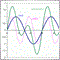| 23 Sep 2019 at 15:02

While compiling TestLibrary.mq4 there are 2 error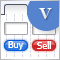Graphical Interfaces V: The List View Element (Chapter 2)
In the previous chapter, we wrote classes for creating vertical and horizontal scrollbars. In this chapter, we will implement them. We will write a class for creating the list view element, a compound part of which will be a vertical scrollbar.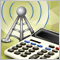Calculator of signals
The calculator of signals operates directly from the MetaTrader 5 terminal, which is a serious advantage, since the terminal provides a preliminary selection and sorts out signals. This way, users can see in the terminal only the signals that ensure a maximum compatibility with their trading accounts.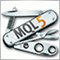Universal Expert Advisor: Trading in a Group and Managing a Portfolio of Strategies (Part 4)
In the last part of the series of articles about the CStrategy trading engine, we will consider simultaneous operation of multiple trading algorithms, will learn to load strategies from XML files, and will present a simple panel for selecting Expert Advisors from a single executable module, and managing their trading modes.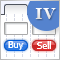Graphical Interfaces IV: the Multi-Window Mode and System of Priorities (Chapter 2)
In this chapter, we will extend the library implementation to the possibility of creating multi-window interfaces for the MQL applications. We will also develop a system of priorities for left mouse clicking on graphical objects. This is required to avoid problems when elements do not respond to the user's actions.